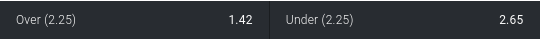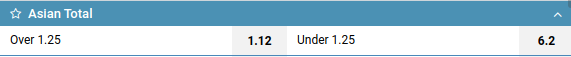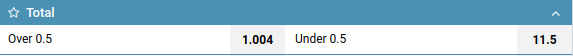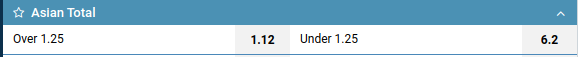# What does Asian total mean in betting?

Asian Total is a type of bet on Total, which is split into two Totals. It can be distinguished by the numerical parameter of Total, which must be a multiple of 0.25. For example “Total Under 2.75” or “Total Over 0.25”.

For example:About Total and what types it happens, we described in our article “Total odds in sports betting”.

Next, for a better understanding, we will consider the Asian total goals for football.

## How is Asian total calculated?

When calculating the Asian Total is divided into two: Total with “Parameter - 0.25” and Total with “Parameter + 0.25”, where Parameter is the numerical value of the total.

For example, “Asian Total 1.75 Over” means the division of the bet into two Totals: “Total 1.5 Over” and “Total 2 Over”. “1.75” in the “Asian total 1.75 over” bet is a numerical Parameter (1.75-0.25 = 1.5 and 1.75 + 0.25 = 2).

Here's another example:Consider a bet on “Over 1.25”.

To calculate the Total must be divided into two: “Over (1.25-0.25)” and “Over (1.25+0.25)”.

We get two bets: “Over 1” with odds 1.12 and “Over 1.5” with the same odds 1.12. Do not confuse the received bets with three-way bets, in case of hitting the total there is a refund.

Distribution of winnings also occurs separately for each of these bets, if one wins and the other loses - the win will be credited only for the won bet. The Asian Total bet amount will be divided into equal parts and distributed over the two bets received.

## Examples of calculating bets on Asian Total

Let's say we bet \$100 on “Over 1.25”.
Let's look at a few examples of the final score in the match and see the results of our bet “Over 1.25”. Recall that the “Over 1.25” bet is divided into 2 bets: “Over 1” and “Over 1.5”, each of which takes half of the total bet of \$100, which means \$50 each.

• 0-0: None of the bets won. Therefore, the \$100 we bet on “Over 1.25” we lost.
• 1-0: “Over 1” - there is a refund, so exactly 1 goal was scored (exact hit in the total), “Over 1.5” - a loss (less than 1.5 goals scored). Refunded \$50 with a bet of \$100.
• 2-0: “Over 1” - win (more than 1 goal was scored), “Over 1.5” - win (more than 1.5 goals were scored). We won at the “Over 1.25” bet completely.

Thus, when betting on Asian Total, we can lose, return half of the bet, or win.

## Asian Individual Total

This is the same Asian Total, but for the team / player. For example below we see Individual Asian Total for the 1st team:## What is the difference between Total and Asian Total

The most important difference between Asian total and simple total is in the numerical parameter of the bet. Asian total has a parameter multiple of 0.25 and is split into two totals.

European total:Asian total:## Different designations of the Asian Total in the lines

Bookmakers display Asian Totals in their Line in different ways, here are some examples:Dafabet bookmaker often uses this form of notation, in which “Over 2.5,3” means “Asian Total Over 2.75”. In this case, the bookmaker indicated the numerical parameters of the two Totals that make up the bet.Pinnacle bookmaker often does not separate Asian and simple (European) totals, but records everything in one group, the only difference here is the numerical Total Parameter, multiple of 0.25.

Asian total is a very common type of bets for many strategies, which has its own calculation features that you need to know. Quite often, this type of bet creates arbitrage situations, which makes it even more valuable.